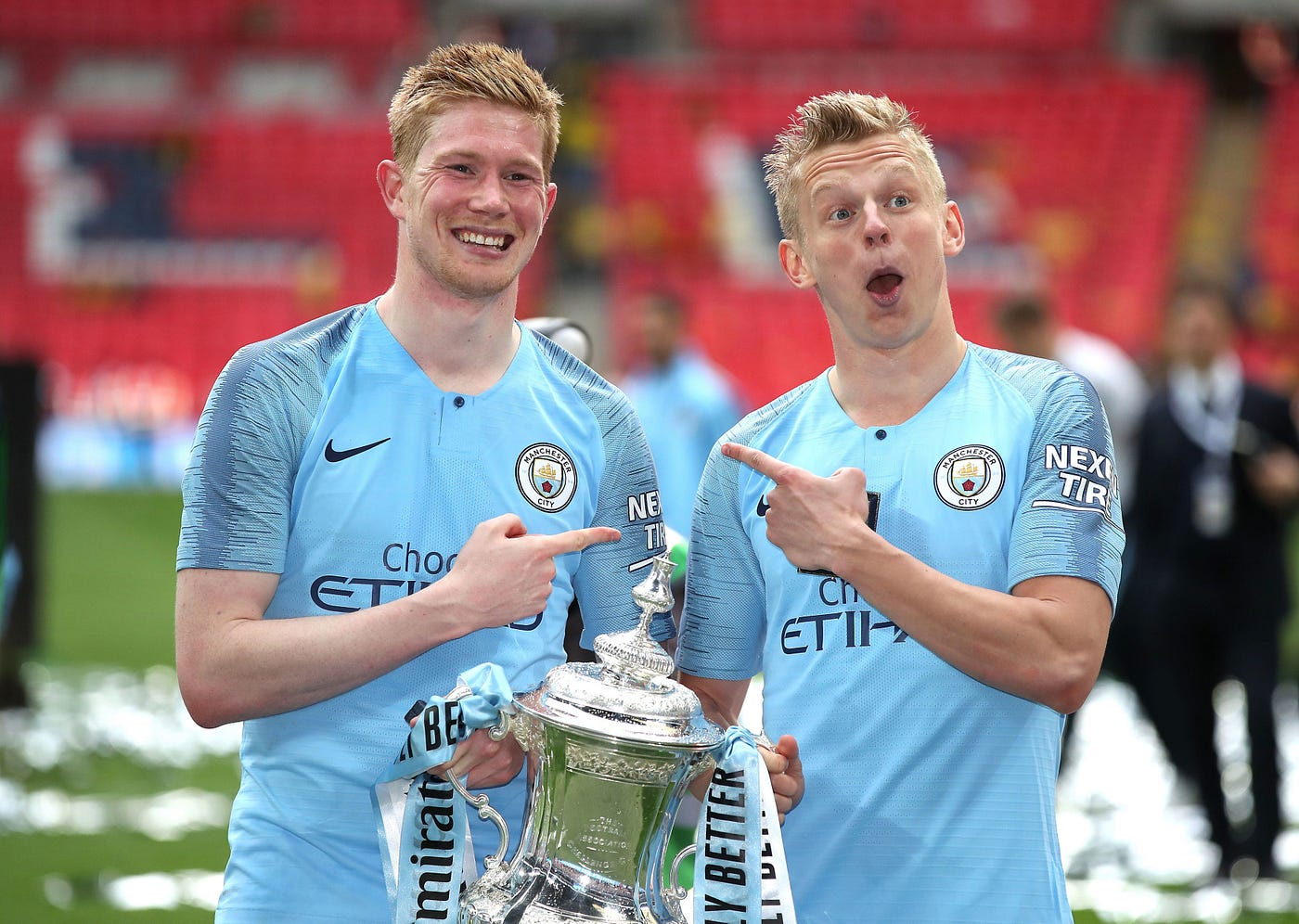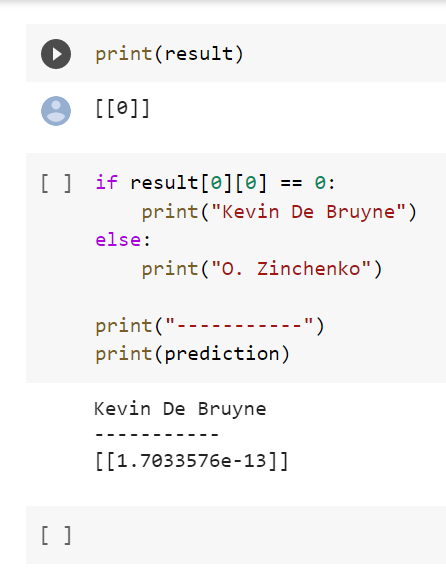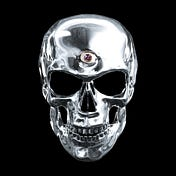# Learning Machines With Me (Part Three.)

A series where I attempt to not only understand, myself, but also do fun things with machine learning — with strangers on the Internet.Today, we’re going to train a computer to analyze pictures

Today’s block in learning machines with me is a bit of a fun exercise. There’s no inherent analysis value however it is fun and extensions of it exist that are important.

Today, we’re going to build a neural network (what!) to analyze pictures and be able to predict/classify the image. This is really a huge step in machine learning as we are basically coding a computer to see and classify images like we do. It’s super fun and at the end, you’ll get to know what neural networks and make one that can classify two things of your liking!

Just like before, here is how to approach this guide:

All you have to do is learn some of the basic level things that are occurring and then, download the dataset and simply run the code! After that, feel free to play around with the code as you see fit and try to make a better model!

In this third part of the series, we’re going to be building a neural network.

Simply put, we’re going to train a computer to find the difference between a Kevin De Bruyne and Oleksandr Zinchenko. These two have been debated to be very similar.

Here have a look:De Bruyne and Zinchenko OR Zinchenko and De Bruyne? Which one is it?

Of course, we can find some interesting features about the two to be able to discriminate the two but can a computer? How accurate can it really be?

While at first, it’s purely fun, extensions of this reach into a branch of machine learning called computer vision. Computer vision seeks to reach and analyze pictures and videos. This technology is at the heart of what’s driving tracking players through video like shown here:

As such, while being fun, it also offers opportunities to develop important technologies in the field of computer vision.

Okay now to explaining how a neural network works.

Neural network? How? You’ve only heard that name on the TV or on YouTube. Aren’t we imitating our brains with a computer? We’re going to break the matrix!

It’s not as complicated as it might seem — on the contrary, it’s pretty intuitive.

A basic neural network is simply a system where we have a single neuron. A ‘neuron’ is a place that takes inputs and transforms/analyzes — another word, is activates — those inputs into a output. It looks like this:

Let’s say we want to analyze relationships between height, weight, age, and grades. Here, we’d input the height, weight, and age into our neuron. Now, all these inputs have a relationship with the neuron.

Those relationships are defined by weights. Maybe height and weight are more important than age at determining a letter grade and for that reason, they get higher weights. These weights is how a neural network learns — these weights help guide the network to learn what’s important and what’s not and how to utilize these things.

In the neuron, what’s happening is something called a weighted sum. In this, the inputs are multiplied by their weights and added together. Here’s an example:

`(Height in cm* Weight of Height) + (Weight in kg* Weight of Weight) + (Age in years * Weight of Age) = Weighted Sum(190 * 0.6) + (90*0.2) + (19*0.2) = 135.8`

That’s the weighted sum of the inputs and then the neuron puts the weighted sum into something called an activation function.

An activation function is a way for making the weighted sum make some sense in the context of the system. For example, the simplest activation function is a threshold function:

So for example, let theta — that’s the circle with a line through it — have a value of 120.

Any values above 120 immediately get assigned the value of 1 — let’s say getting the letter grade of A — while any value below 120 gets the value of 0 which means not getting the letter grade of A.

Our weighted sum had a value of 135.8 which, with this function, means we are predicted to have a letter grade of A with height of 190 cm, weight of 90 kg, and being 19 years of age.

These activations functions are very important as they dictate how neurons interact with each other and additionally, are the final thing a neural network before spitting an output that we understand.

Neural networks then compare the predicted output to the actual output and backpropagate — fancy term for working backwards — from the error to fix the weights of the relationships between inputs and neurons.

There’s a lot of other things working in the background like gradient descents, backpropagation, forward propagation, and cost functions but that is really technical details that we don’t need.

This is a great link to interact with a neural network

# Building Our Neural Network

Alright, let’s actually build our neural network.

You can fork my open repository to get everything in one folder: https://github.com/abhiamishra/FindingDeBruyne

OR, follow these steps:

Here is the training dataset. Download the entire folder and do not change the name of the sub-folders: TrainingDataSet

Here is the validation dataset. Download the entire folder and do not change the name of the sub-folders: ValidationDataSet

Here is the real test dataset. Download the entire folder and do not change the name of the folders: RealDataSet

For this project, you will need Jupyter Notebook. There are tons of tutorials online to get that running so get that installed. Then, download this .ipynb file: FindingDeBruyne

Next up, please put the training, validation, and real dataset inside a folder. Inside the same folder, put the .ipynb file.

Then name the training dataset to ‘training_set’, name the validation dataset to ‘test_set’, name the real test dataset to ‘real_test’.

How to Get Your Predictions

Run all the cells and then when you get to your predictions, do the following.

Simply go in your real_test folder and pick an image you want to get a prediction of and place the name of file where I’ve bolded here in the code:

Add any image to your real_test folder, save the folder, and re-run this cell of code with the updated name to see the new prediction!

`import numpy as npfrom tensorflow.keras.preprocessing import imagetest_image = image.load_img('NAME_OF_FILE_IN_REAL_TEST_FOLDER.jpg', target_size=(64,64))test_image = image.img_to_array(test_image)test_image = np.expand_dims(test_image, axis=0)result = cnn.predict_classes(test_image)prediction = cnn.predict_proba(test_image)training_set.class_indices`

Run the cells below this piece of code in the Jupyter Notebook and you’ll see what it predicts, what that prediction means (KdB or Zinchenko), and the actual probability of the prediction so that you can see what the model specifically thought.The model predicting who is a Kevin De Bruyne and what is a Oleksandr Zinchenko

# Some Interactivity

To see if you can better the model, see if adding more runs of training makes the model better or does it make the accuracy stay the same by changing the following code:

`cnn.fit(x=training_set, validation_data = test_set, epochs=INSERT A NUMBER GREATER THAN 0)`

Try changing the activation functions. Here, try out an activation function known as hyperbolic tangent:

`#Convolutioncnn.add(tf.keras.layers.Conv2D(filters=32, kernel_size=3, strides=(2,2), activation = 'tanh', input_shape = [64, 64, 3]))`

Please feel free to reach out to me on Twitter if you have any questions. Leave any comments on how to improve!

--

--## Abhishek Amol Mishra

Writer. Aspiring Computer & Data Scientist. “We’re made of star stuff. We are a way for the cosmos to know itself “ — Carl Sagan